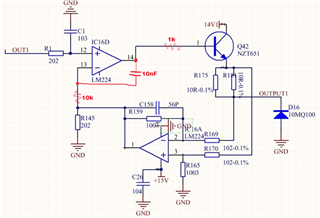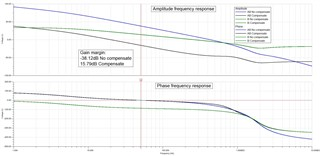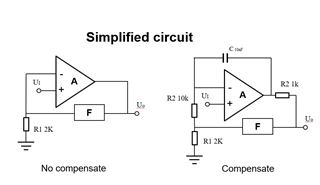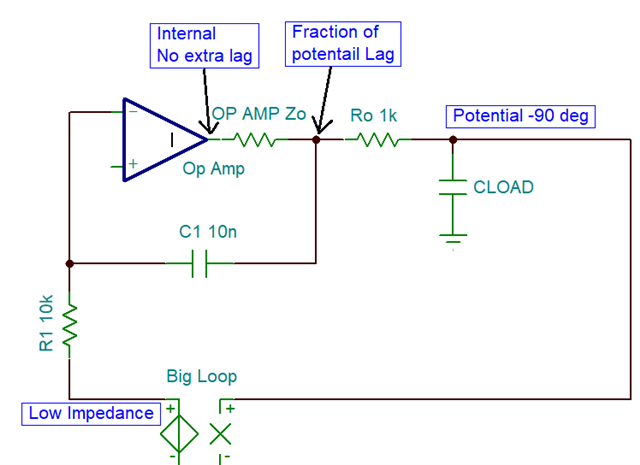If you have a related question, please click the "Ask a related question" button in the top right corner. The newly created question will be automatically linked to this question.

# LM224: What is the principle of this compensation circuit？

Part Number: LM224

Hi engineers of TI, I have a question about Op amp compensation and open loop transfer function：

There is a 0~10V input， 0~20mA output CCS as follows, the circuit is unstable at first,(especially  when the load is capacitive). Transient response simulation results show that the output is oscillating.

So some compensate component(show as red line, include two resistor and one capacitance)be  added to make the loop stable.After compensate, Transient response simulation results show that the output is stabled.Open-loop amplitude-frequency and phase-frequency simulation results show as below, the result show that gain margin of open loop  is -38.12dB at first and15.79 after compensate.So, What is the principle of the compensation circuit?

Because I just wanna figure out the effect of compensate part, I simplified the circuit (remove the transistor and simplify the differential amplifier to F), Simplified circuit show as follows.

What is the open loop transfer function(AF) of the Initial circuit and compensated circuit ？• Stanley,

The basic idea is to pass low frequencies through the entire full loop to perform the desired task. At higher frequency (>Fc of new 10k 10nF), the feedback will switch to a smaller loop, output back to inverting input (bypassing the rest of the loop). This smaller loop does not have gain and has less phase loss than the full loop.

• I can understand the 10nF supply a smaller loop for high frequency signal and the phase loss is less than full loop which avoid the risk of negative phase margin, but it's seem like only a 10nF can achieve that purpose, what's the function of 10k and 1k??

• Morning Stanley

As a general principal if your load (the transistor here) looks capacitive you might want to isolate that from the op amp output stage. That 1kohm seems high, I would be using a 100ohm perhaps to avoid putting another pole in the low frequency loop that is in band so to speak.

The 10kohm? that gives a better feedback path for the overall loop I suspect.

• Stanley,

The concept in picture form.The 1k in conjunction with the op amp Zo reduces the potential phase loss due to a capacitive load at output pin.

The 10k increases the impedance for the big loop path. If the gain of Big loop is 1 then the frequency where the short loop (C1) become dominant is 1/(2piRC) = 1.6kHz .  If big loop gain is >1 the "switch" frequency will be higher. If big loop gain <1  the "switch" frequency will be lower.